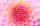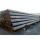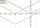# Reciprocal equation 2

Solve this equation:

x + 5/x - 6 = 4/11

Result

x1 =  5.445
x2 =  0.918

#### Solution:Checkout calculation with our calculator of quadratic equations.Leave us a comment of example and its solution (i.e. if it is still somewhat unclear...):Be the first to comment!#### To solve this verbal math problem are needed these knowledge from mathematics:

Looking for help with calculating roots of a quadratic equation? Do you have a linear equation or system of equations and looking for its solution? Or do you have quadratic equation?

## Next similar examples:

1. Solve 3Solve quadratic equation: (6n+1) (4n-1) = 3n2
2. Variation equationSolve combinatorics equation: V(2, x+8)=72
3. VariableFind variable P: PP plus P x P plus P = 160
4. EquationEquation ? has one root x1 = 8. Determine the coefficient b and the second root x2.
5. DiscriminantDetermine the discriminant of the equation: ?Find the roots of the quadratic equation: 3x2-4x + (-4) = 0.
7. RootsDetermine the quadratic equation absolute coefficient q, that the equation has a real double root and the root x calculate: ?
8. Evaluation of expressionsIf a2-3a+1=0, find (i)a2+1/a2 (ii) a3+1/a3
9. TubesIron tubes in the warehouse are stored in layers so that each tube top layer fit into the gaps of the lower layer. How many layers are needed to deposit 100 tubes if top layer has 9 tubes? How many tubes are in bottom layer of tubes?Quadratic equation ? has roots x1 = 80 and x2 = 78. Calculate the coefficients b and c.
11. Cinema 4In cinema are 1656 seats and in the last row are 105 seats , in each next row 3 seats less. How many are the total rows in cinema?If 5x + x² > 100, then x is notHow many solutions has the equation ? in the real numbers?If the square root of 3m2 +22 and -x = 0, and x=7, what is m?From how many elements we can create 990 combinations 2nd class without repeating?How many elements can form six times more combinations fourth class than combination of the second class?We want to prove the sentence: If the natural number n is divisible by six, then n is divisible by three. From what assumption we started?